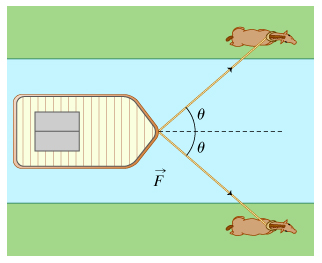# Problem: Two workhorses tow a barge along a straight canal. Each horse exerts a constant force of magnitude F, and the tow ropes make an angle θ with the direction of motion of the horses and the barge.  Each horse is traveling at a constant speed v(a) How much work W is done by each horse in a time t? Express the work in terms of the quantities given in the problem introduction.(b) How much power P does each horse provide? Express your answer in terms of the quantities given in the problem introduction.

###### FREE Expert Solution

Word done:

$\overline{){\mathbf{W}}{\mathbf{=}}{\mathbf{F}}{\mathbf{·}}{\mathbf{d}}}$

Distance:

$\overline{){\mathbf{d}}{\mathbf{=}}{\mathbf{v}}{\mathbf{·}}{\mathbf{t}}}$

Power:

$\overline{){\mathbf{P}}{\mathbf{=}}\frac{\mathbf{W}}{\mathbf{t}}}$

(a)

Force applied on the barge by each horse = (F cos θ)

99% (342 ratings)###### Problem Details

Two workhorses tow a barge along a straight canal. Each horse exerts a constant force of magnitude F, and the tow ropes make an angle θ with the direction of motion of the horses and the barge.  Each horse is traveling at a constant speed v(a) How much work W is done by each horse in a time t? Express the work in terms of the quantities given in the problem introduction.

(b) How much power P does each horse provide? Express your answer in terms of the quantities given in the problem introduction.